# Orthogonal Coordinate Systems - Cartesian, Cylindrical, and Spherical

### Base Vectors

In a  three-dimensional space, a point can be located as the intersection of three surfaces. The three surfaces are described by

u1 = constant
u2 = constant
u3 = constant

u1, u2, and u3 need not all be lengths as shown in the table below.

 u1 u2 u3 Cartesian Coordinate System x y z Cylindrical Coordinate System r φ z Spherical Coordinate System R θ φ

If these three surfaces (in fact, their normal vectors) are mutually perpendicular to each other, we call them orthogonal coordinate system.

Cartesian Coordinate System:

In Cartesian coordinate system, a point is located by the intersection of the following three surfaces:

• A plane parallel to the y-z plane (x = constant, normal to the x axis, unit vector)
• A plane parallel to the x-z plane (y = constant, normal to the y axis, unit vector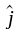)
• A plane parallel to the x-y plane (z = constant, normal to the z axis, unit vector)

This is shown in the figure below.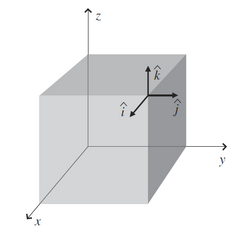Base Vectors:,, andare the unit vectors in the three coordinate directions. They are called the base vectors.

The base vectors meet the following relations: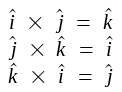Cylindrical Coordinate System:

In cylindrical coordinate systems a point P(r1, θ1, z1) is the intersection of the following three surfaces as shown in the following figure.

• A circular cylindrical surface r = r1
• A half-plane containing the z-axis and making angle φ = φ1 with the xz-plane
• A plane parallel to the xy-plane at z = z1.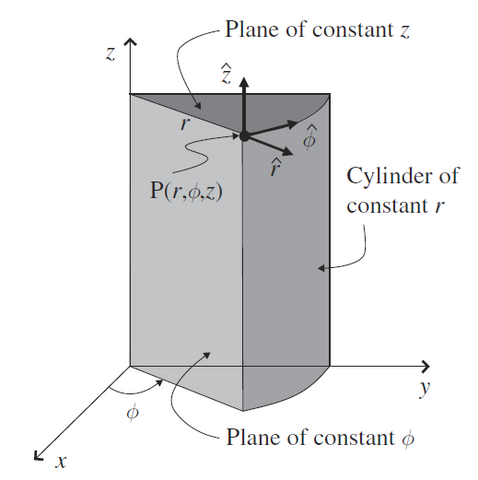Base Vectors:

• The base vectorat P is perpendicular to the cylindrical surface of constant r1.
• The base vectorat P is perpendicular to the half-plane surface of constant φ1 and tangential to the cylindrical surface of constant r1.
• The base vectoris perpendicular to the plane of constant z1.

The base vectors meet the following relations: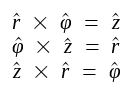Spherical Coordinate System:

A point P(R1θ1, φ1) in spherical coordinates is located at the intersection of the following three surfaces:

• A spherical surface centered at the origin with a radius R = R1 (sphere of constant R)
• A right circular cone with its apex at the origin, its axis coinciding with the +z axis, and having a half-angle θθ1 (cone of constant θ)
• A half-plane containing the z-axis and making an angle φφ1 with the xz-plane (plane of constant φ)

This is shown below.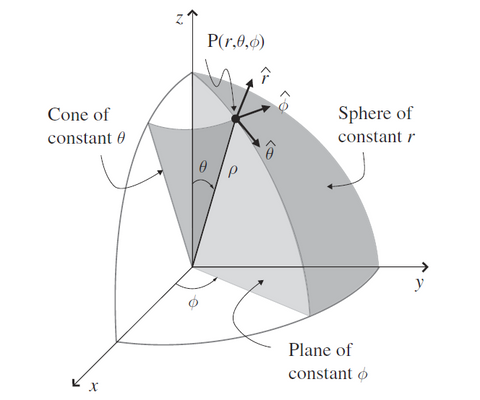Base Vectors:

• The base vectorat P is radial from the origin and is perpendicular to the sphere of constant R = R1
• The base vectorat P is perpendicular to the cone of constant θθ1
• The base vectorat P is per perpendicular to the plane of constant φφ1

The base vectors meet the following relations: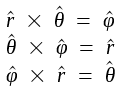### Metric Coefficients

In vector calculus and electromagnetics work we often need to perform line, surface, and volume integrals. In these cases, we need to find the differential length change (dl), differential area change (ds), and differential volume change (dv) in the chosen coordinate system.Line Integral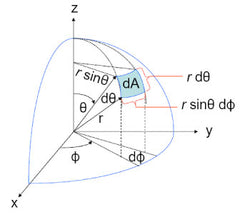Surface IntegralVolume Integral

Cartesian coordinate system is length based, since dx, dy, dz are all lengths. However, in other curvilinear coordinate systems, such as cylindrical and spherical coordinate systems, some differential changes are not length based, such as dθ, .

Thus, we need a conversion factor to convert (mapping) a non-length based differential change (dθ, , etc.) into a change in length dl as shown below.

dli = hdui

where i = (1, 2, or 3), hi is called the metric coefficient and may itself be a function of  u1, u2, u3.

u1, u2, u3 are coordinate axes in the chosen system, and correspond to x, y, z in Cartesian coordinate, r, φ, z in cylindrical coordinate, and Rθφ in spherical coordinate system.

Examples:

In the two-dimensional polar coordinates (u1, u2) = (r, φ), a differential change (= du2) corresponds to a differential length-change dl2 = r (h2 = r = u1).

In Cartesian coordinate systems, since dx, dy, dz are already length based, h1 = h2 = h3 = 1.

### Differential Lengths

From the above discussion, we can see the differential length changes dl1, dl2, dl3 are:

dl1 = h1 du1
dl2 = h2 du2
dl3 = h3 du3

where h1, h2, h3 may be functions of u1, u2, and u3.

A directed differential length-change dl in an arbitrary direction can be written as the vector sum of the component length-changes: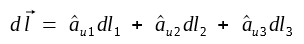orwhere au1, au2, au3 are the base vectors.

### Differential AreaIn general orthogonal curvilinear coordinates the differential area ds1 (dA as shown in the figure above) normal to the unit vector au1 is:

ds1 = dl2 dl3

thus

ds1 = h2 h3 du2 du3

Similarly, the differential areas normal to unit vectors au2, au3 are:

ds2 = h1 h3 du1 du3

ds3 = h1 h2 du1 du2

For example, in Cartesian coordinate system:

dsx = dy dz

dsy = dz dx

dsz = dx dy

where h1 = h2 = h3 = 1.

### Differential Volume

The differential volume dv formed by differential coordinate changes du1, du2, and du3 in directions au1, au2, and au3, respectively, is (dl1 dl2 dl3):

dv = dl1 dl2 dl3 = h1 h2 h3 du1 du2 du3

Cartesian Coordinates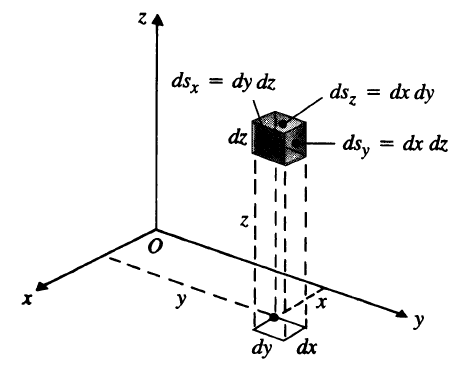Cylindrical Coordinates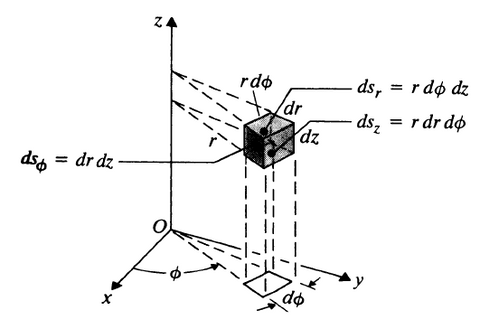Spherical Coordinates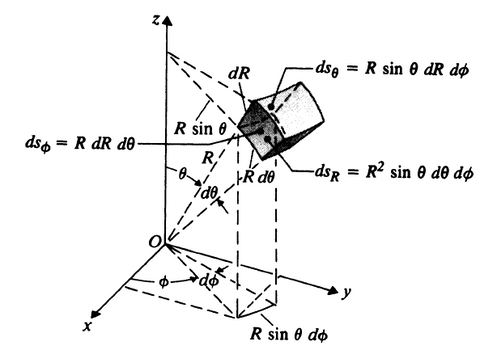For convenience, the base vectors, metric coefficients, differential lengths, differential areas, and differential volume are listed in the following table.

 Cartesian Coordinates (x, y, z) Cylindrical Coordinates (r, φ, z) Spherical Coordinates (R, θ, φ) Base Vectors au1au2au3Metric Coefficients h1 1 1 1 h2 1 r R h3 1 1 R sinθ Differential Length dl1 dx dr dR dl2 dy r dφ R dθ dl3 dz dz R sinθ dφ Differential Area ds1 dy dz r dφ dz R2 sinθ dθ dφ ds2 dx dz dr dz R sinθ dR dφ ds3 dx dy r dr dφ R dR dθ Differential Volume dv dx dy dz r dr dφ dz R2 sinθ dR dθ dφ